# CORPORATE FINANCE REVIEW FOR FIRST QUIZ Aswath Damodaran

• Slides: 23CORPORATE FINANCE REVIEW FOR FIRST QUIZ Aswath Damodaran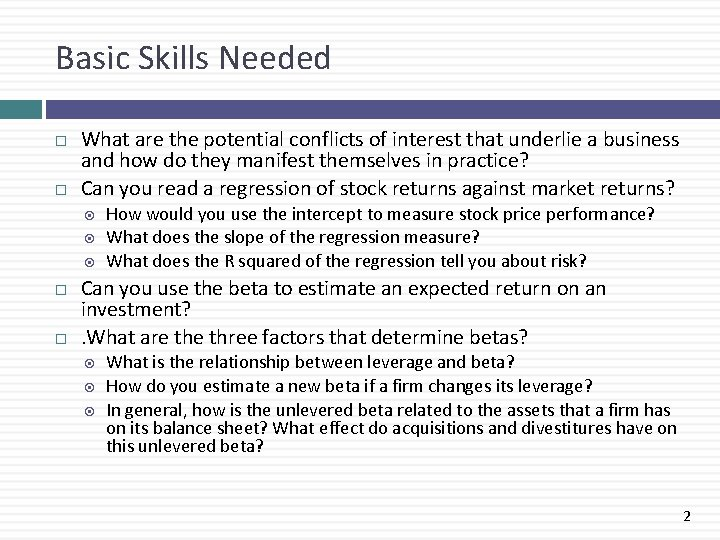Basic Skills Needed What are the potential conflicts of interest that underlie a business and how do they manifest themselves in practice? Can you read a regression of stock returns against market returns? How would you use the intercept to measure stock price performance? What does the slope of the regression measure? What does the R squared of the regression tell you about risk? Can you use the beta to estimate an expected return on an investment? . What are three factors that determine betas? What is the relationship between leverage and beta? How do you estimate a new beta if a firm changes its leverage? In general, how is the unlevered beta related to the assets that a firm has on its balance sheet? What effect do acquisitions and divestitures have on this unlevered beta? 2Corporate governance. . Use common sense… Assume that you are currently a stockholder in a firm that is the target of a leveraged buyout. From the perspective of conflicts of interests between you and management, which of the following should concern you the most about this transaction? The fact that the company will go private after the transaction The possibility that equity investors in the LBO can make huge returns The fact that the managers of your firm are also part of the acquisition team The use of a disproportionate amount of debt to fund the transaction None of the above We usually see the stock prices of the target firm in an LBO jump on the announcement of an LBO. This can be viewed as evidence that Markets are inefficient Markets are efficient Cannot tell without more information 3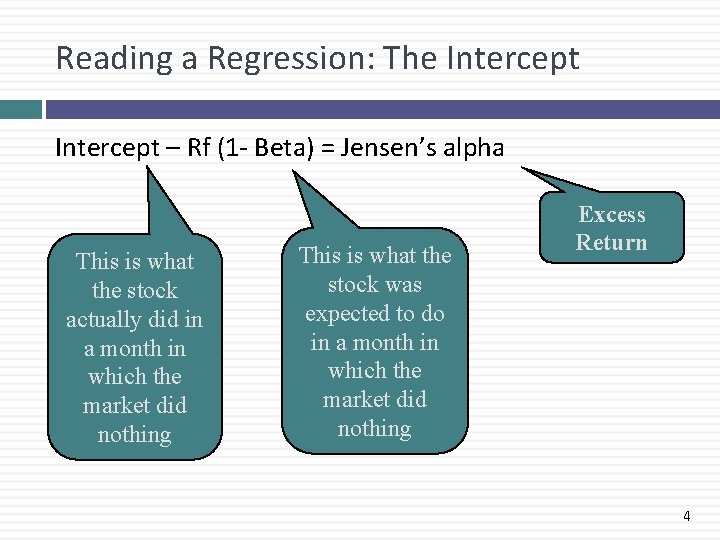Reading a Regression: The Intercept – Rf (1 - Beta) = Jensen’s alpha This is what the stock actually did in a month in which the market did nothing This is what the stock was expected to do in a month in which the market did nothing Excess Return 4Key concepts to keep in mind about the intercept It is the difference between the two (the intercept and the riskfree rate (1 -beta)) that matters The intercept and the riskfree rate have to be stated in the same terms – if one is monthly, the other has to be monthly as well. 5An Example You have run a regression of returns of Devonex, a machine tool manufacturer, against the S&P 500 Index using monthly returns over the last 5 years and arrived at the following regression: Return. Devonex = - 0. 20% + 1. 50 Return S&P 500 If the stock had a Jensen’s alpha of +0. 10% (on a monthly basis) over this period, estimate the monthly riskfree rate during the last 5 years. 6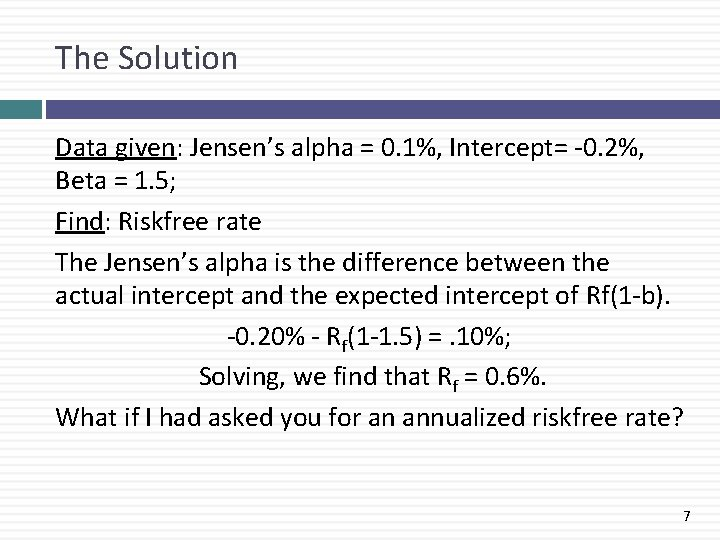The Solution Data given: Jensen’s alpha = 0. 1%, Intercept= -0. 2%, Beta = 1. 5; Find: Riskfree rate The Jensen’s alpha is the difference between the actual intercept and the expected intercept of Rf(1 -b). -0. 20% - Rf(1 -1. 5) =. 10%; Solving, we find that Rf = 0. 6%. What if I had asked you for an annualized riskfree rate? 7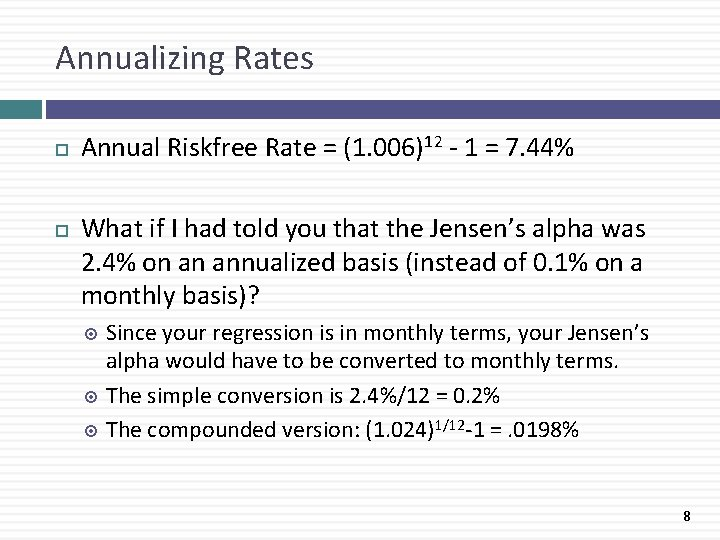Annualizing Rates Annual Riskfree Rate = (1. 006)12 - 1 = 7. 44% What if I had told you that the Jensen’s alpha was 2. 4% on an annualized basis (instead of 0. 1% on a monthly basis)? Since your regression is in monthly terms, your Jensen’s alpha would have to be converted to monthly terms. The simple conversion is 2. 4%/12 = 0. 2% The compounded version: (1. 024)1/12 -1 =. 0198% 8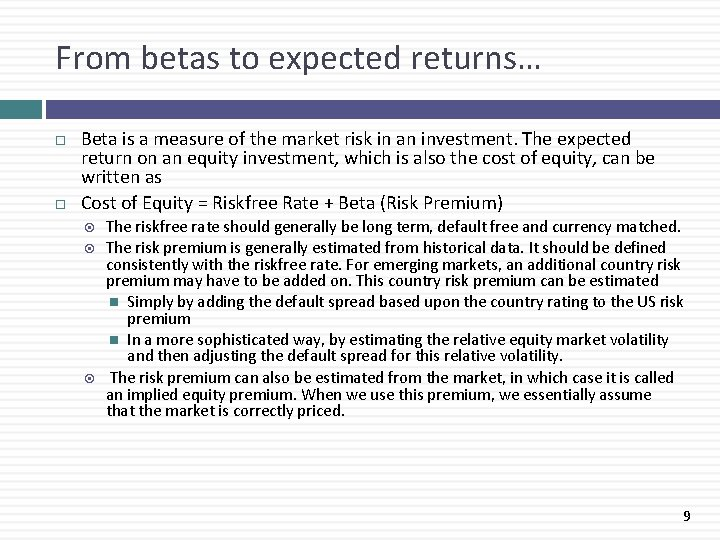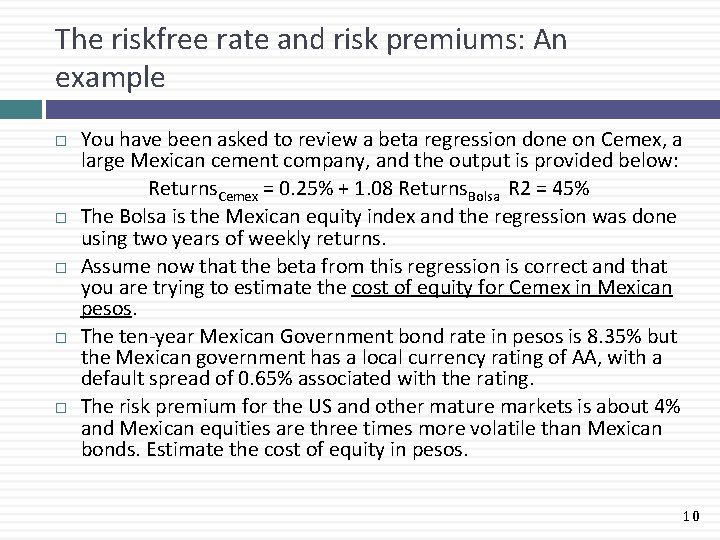The riskfree rate and risk premiums: An example You have been asked to review a beta regression done on Cemex, a large Mexican cement company, and the output is provided below: Returns. Cemex = 0. 25% + 1. 08 Returns. Bolsa R 2 = 45% The Bolsa is the Mexican equity index and the regression was done using two years of weekly returns. Assume now that the beta from this regression is correct and that you are trying to estimate the cost of equity for Cemex in Mexican pesos. The ten-year Mexican Government bond rate in pesos is 8. 35% but the Mexican government has a local currency rating of AA, with a default spread of 0. 65% associated with the rating. The risk premium for the US and other mature markets is about 4% and Mexican equities are three times more volatile than Mexican bonds. Estimate the cost of equity in pesos. 10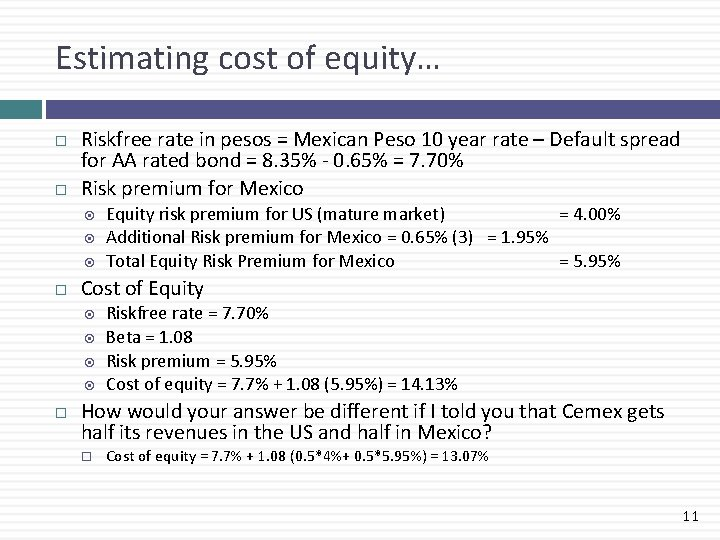Estimating cost of equity… Riskfree rate in pesos = Mexican Peso 10 year rate – Default spread for AA rated bond = 8. 35% - 0. 65% = 7. 70% Risk premium for Mexico Cost of Equity risk premium for US (mature market) = 4. 00% Additional Risk premium for Mexico = 0. 65% (3) = 1. 95% Total Equity Risk Premium for Mexico = 5. 95% Riskfree rate = 7. 70% Beta = 1. 08 Risk premium = 5. 95% Cost of equity = 7. 7% + 1. 08 (5. 95%) = 14. 13% How would your answer be different if I told you that Cemex gets half its revenues in the US and half in Mexico? Cost of equity = 7. 7% + 1. 08 (0. 5*4%+ 0. 5*5. 95%) = 13. 07% 11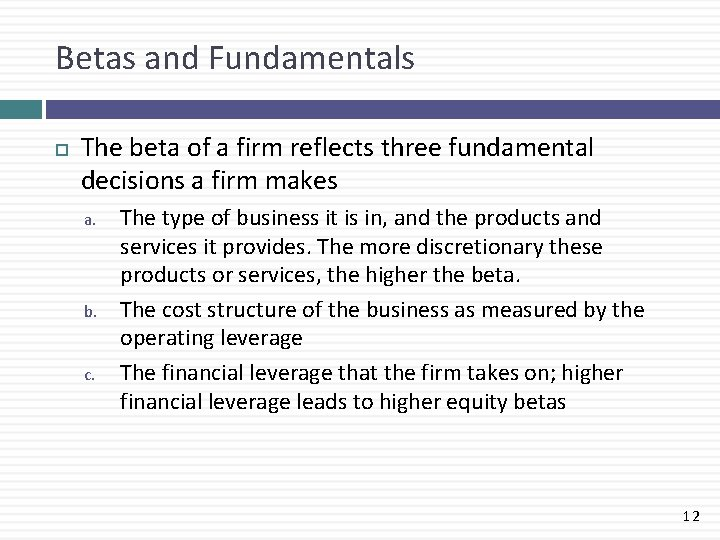Betas and Fundamentals The beta of a firm reflects three fundamental decisions a firm makes a. b. c. The type of business it is in, and the products and services it provides. The more discretionary these products or services, the higher the beta. The cost structure of the business as measured by the operating leverage The financial leverage that the firm takes on; higher financial leverage leads to higher equity betas 12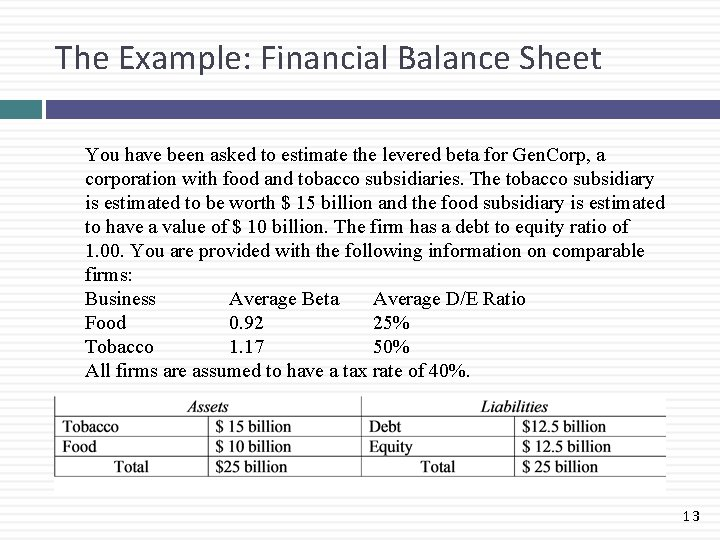The Example: Financial Balance Sheet You have been asked to estimate the levered beta for Gen. Corp, a corporation with food and tobacco subsidiaries. The tobacco subsidiary is estimated to be worth \$ 15 billion and the food subsidiary is estimated to have a value of \$ 10 billion. The firm has a debt to equity ratio of 1. 00. You are provided with the following information on comparable firms: Business Average Beta Average D/E Ratio Food 0. 92 25% Tobacco 1. 17 50% All firms are assumed to have a tax rate of 40%. 13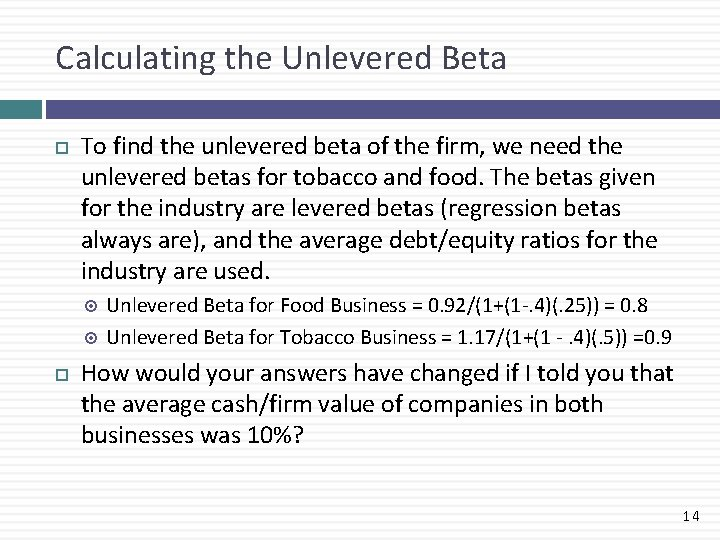Calculating the Unlevered Beta To find the unlevered beta of the firm, we need the unlevered betas for tobacco and food. The betas given for the industry are levered betas (regression betas always are), and the average debt/equity ratios for the industry are used. Unlevered Beta for Food Business = 0. 92/(1+(1 -. 4)(. 25)) = 0. 8 Unlevered Beta for Tobacco Business = 1. 17/(1+(1 -. 4)(. 5)) =0. 9 How would your answers have changed if I told you that the average cash/firm value of companies in both businesses was 10%? 14An Updated Balance Sheet 15The Effects of Leverage Unlevered Beta = 0. 864 Levered Beta for the Firm = 0. 864 (1 + (1 -. 4) (12. 5/12. 5)) = 1. 376 Cost of Equity = 6% + 1. 376 (5. 5%) = 13. 57% 16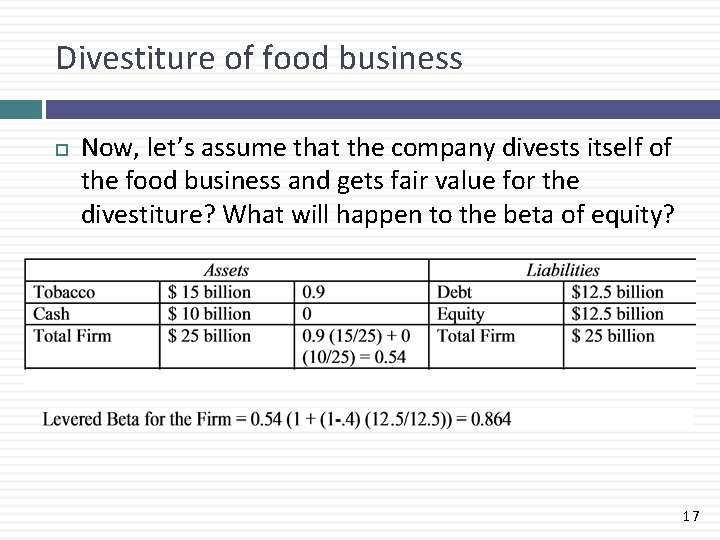Divestiture of food business Now, let’s assume that the company divests itself of the food business and gets fair value for the divestiture? What will happen to the beta of equity? 17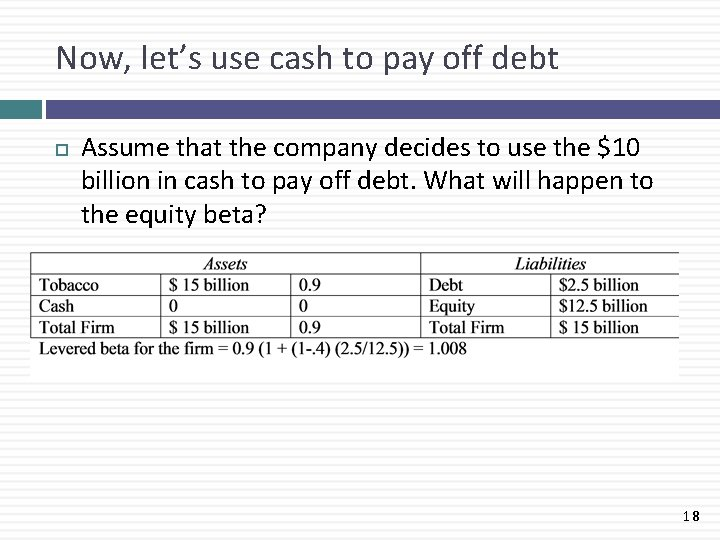Now, let’s use cash to pay off debt Assume that the company decides to use the \$10 billion in cash to pay off debt. What will happen to the equity beta? 18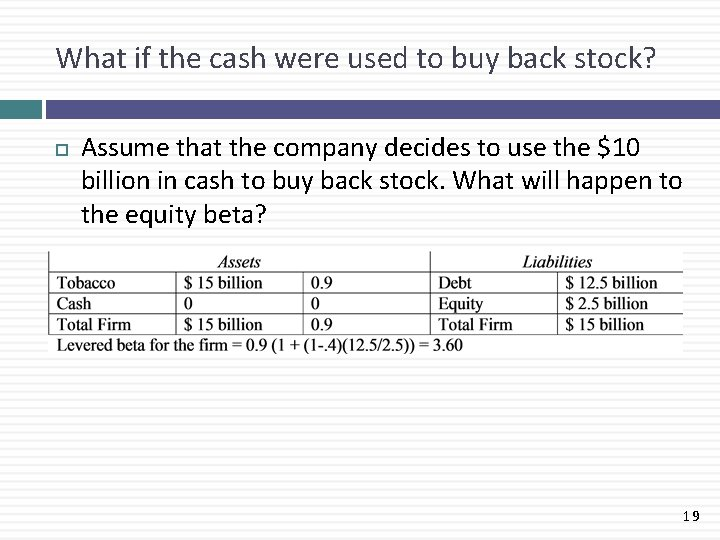What if the cash were used to buy back stock? Assume that the company decides to use the \$10 billion in cash to buy back stock. What will happen to the equity beta? 19What if the cash were used to pay a dividend of \$ 2. 5 billion, pay down debt of \$ 7. 5 billion Assume that the company decides to use the \$2. 5 billion to pay a dividend and the remaining \$7. 5 billion to pay down debt. What will happen to the equity beta? 20What if the cash were used to buy a social media firm for \$ 10 billion Assume that the company decides to use the \$10 billion in cash to buy a social media company. What will happen to the equity beta? (Social media companies have an unlevered beta of 1. 80) 21What if the cash is used plus debt of \$ 5 billion to buy a social firm for \$ 15 billion Assume that the company decides to borrow \$5 billion, and use it with the \$10 billion in cash to buy a social media company for \$15 billion. What will happen to the equity beta? 22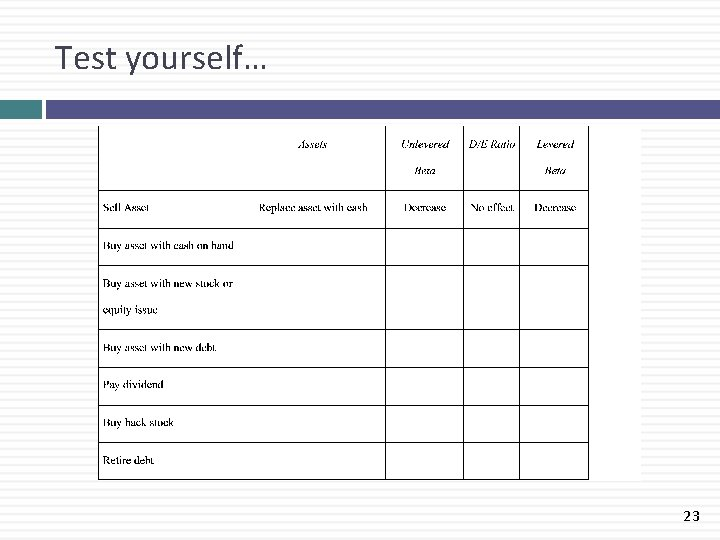Test yourself… 23